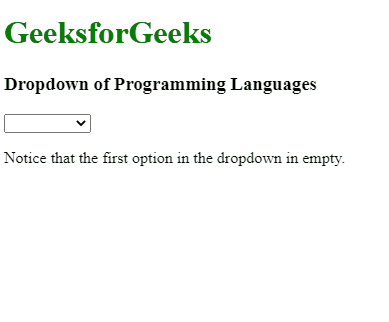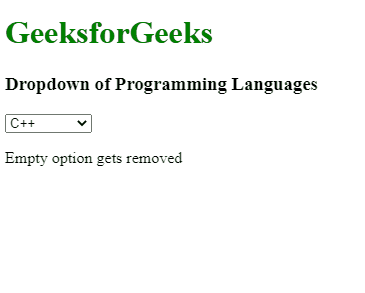# Why does AngularJS include an empty option in select ?

• Last Updated : 01 Aug, 2022

AngularJS is a Javascript framework containing several built-in functionalities, that intend to make the development easier and more efficient for the developers. The dropdown is one of them. A dropdown is a GUI element that enables the user to choose one value from a list of values. When a drop-down is active, a list of values gets displayed to the user, out of which one value can be selected at a time. After a value is selected, the drop-down gets back to its default state. However, while working with the AngularJS dropdown, it was observed that the first option in the select dropdown is always empty. Have a look at the following example for a better understanding.

Example 1: The below example illustrates an empty option in the select menu in AngularJS.

## HTML

 ` ` `<``html``> ` ` `  `<``head``> ` `    ``<``script` `src``= ` `"https://cdnjs.cloudflare.com/ajax/libs/angular.js/1.0.3/angular.js"`  `            ``integrity``= ` `"sha256-ZYJ4XH6kZrqD1MlZWZZmQj6MBe/TvysMc33LPchVuo8="`  `            ``crossorigin``=``"anonymous"``> ` `    `` ` ` ` ` `  `<``body``> ` `    ``<``h1` `style``=``"color:green;"``> ` `        ``GeeksforGeeks ` `    `` ` `    ``<``h3``> ` `        ``Dropdown of Programming Languages ` `    `` ` `    ``<``div` `ng-App``=``"myApp"` `ng-controller``=``"MyCtrl"``> ` `        ``<``select` `ng-model``=``'form.type'` `             ``ng-options``=``'name.val as name.label for name in allNames'``> ` `        `` ` `    `` ` `    ``<``p``> Notice that the first option in the dropdown in empty. ` `    ``<``script``> ` `        ``var myApp = angular.module('myApp', []); ` `        ``function MyCtrl(\$scope) { ` `            ``\$scope.allNames = [{ ` `                ``label: 'C', ` `                ``val: 'C Language' ` `            ``}, { ` `                ``label: 'C++', ` `                ``val: 'C++ Language' ` `            ``}, { ` `                ``label: 'Java', ` `                ``val: 'Java Language' ` `            ``}, { ` `                ``label: 'Python', ` `                ``val: 'Python Language' ` `            ``}, { ` `                ``label: 'JavaScript', ` `                ``val: 'JavaScript Language' ` `            ``}]; ` `        ``} ` `    `` ` ` ` ` `  ``

Output:Explanation and Correction: Generally, a list of options are transferred to ng-option directive. Those options are then displayed to the user in GUI format. However, when some value referenced by the ng-model directive doesn’t exist in the list transferred to the ng-option, then an empty option gets created in the GUI list, which can be seen above example. The easiest way to correct this error is to reference a valid value from the ng-option list. The value referenced must be available on the list. We can reference a valid value using the below code. Here, i will be the index of the value that we want to reference.

`\$scope.form = {type : \$scope.allNames[i].val}; `

Just add this code below the list to remove the empty option from the select. In the example given below, we are modifying the above example, in such a way that the empty option gets removed from the drop-down. We will set the value of i to 1. After doing this, the empty option will disappear and the default selected value will be set to C++.

Example 2: This example illustrates removing the empty option by selecting an initial value in the controller in AngularJS.

## HTML

 ` ` `<``html``> ` ` `  `<``head``> ` `    ``<``script` `src``= ` `"https://cdnjs.cloudflare.com/ajax/libs/angular.js/1.0.3/angular.js"` `            ``integrity``= ` `"sha256-ZYJ4XH6kZrqD1MlZWZZmQj6MBe/TvysMc33LPchVuo8="`  `            ``crossorigin``=``"anonymous"``> ` `    `` ` ` ` ` `  `<``body``> ` `    ``<``h1` `style``=``"color:green;"``> ` `        ``GeeksforGeeks ` `    `` ` `    ``<``h3``> ` `        ``Dropdown of Programming Languages ` `    `` ` `    ``<``div` `ng-App``=``"myApp"` `         ``ng-controller``=``"MyCtrl"``> ` `        ``<``select` `ng-model``=``'form.type'`  `             ``ng-options``=``'name.val as name.label for name in allNames'``> ` `        `` ` `    `` ` `    ``<``p``>Empty option gets removed ` `    ``<``script``> ` `        ``var myApp = angular.module('myApp', []); ` `     `  `        ``function MyCtrl(\$scope) { ` `            ``\$scope.allNames = [{ ` `                ``label: 'C', ` `                ``val: 'C Language' ` `            ``}, { ` `                ``label: 'C++', ` `                ``val: 'C++ Language' ` `            ``}, { ` `                ``label: 'Java', ` `                ``val: 'Java Language' ` `            ``}, { ` `                ``label: 'Python', ` `                ``val: 'Python Language' ` `            ``}, { ` `                ``label: 'JavaScript', ` `                ``val: 'JavaScript Language' ` `            ``}]; ` `            ``\$scope.form = { ` `                ``type: \$scope.allNames.val ` `            ``}; ` `        ``} ` `    `` ` ` ` ``

Output:My Personal Notes arrow_drop_up
Recommended Articles
Page :